# KVPY-SA 2016 Physics Paper with Solutions

KVPY SA 2016 Physics paper solutions are available here. These solved questions will boost the candidates’ confidence and help to score well in the KVPY exam. All the solutions are solved by subject matter experts by using the simplest approach.
The objective of the KVPY is to motivate students to pursue a career in science research. The applicants are advised to practise previous years’ question papers in order to clear the exam. Students can easily access the answer key for KVPY SA Physics 2016 paper from our website and download in PDF format for free from the given link below.

### KVPY SA 2016 - Physics

Question 1: A person walks 25.0° north of east for 3.18 km. How far would she have to walk due north and then due east to arrive at the same location?

1. a. towards north 2.8 km and towards east 1.34 km
2. b. towards north 2.11 km and towards east 2.11 km
3. c. towards north 1.25 km and towards east 1.93 km
4. d. towards north 1.34 km and towards east 2.88 km

Solution: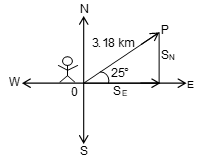Initial position is at 0

SE = distance towards east

SN = distance towards north

Clearly from trigonometry, we can see that SN can be written as

SN = 3.18 Km x sin25°

And SE = 3.18 Km x cos 25°

⇒ SN = 3.18 x 0.42

SN = 1.34 Km (towards North) {sin 25° = .42}

⇒ SE = 3.18 x 0.9

SE = 2.88 Km (towards East) {cos 25° = 0.9}

Question 2: The length and width of a rectangular room are measured to be 3.95±0.05 m and 3.05±0.05 m, respectively, the area of the floor is

1. a. 12.05±0.01 m2
2. b. 12.05±0.005 m2
3. c. 12.05±0.34 m2
4. d. 12.05±0.40 m2

Solution:

Given, that length of the rectangular room (l) = 3.95 ± 0.05 m

Width of rectangular room (b) = 3.05 ± 0.05m

Area of rectangle (A)= length × width

= 3.95 × 3.05

= 12.0475

= 12.048 (By rounding off)

= 12.05 (up to 4 significant figure)

So, by error analysis method the error in area –

Relative error in area = relative error in length + relative error in width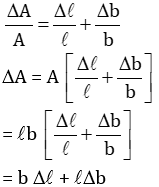= 3.05 × 0.05 + 3.95 × 0.05

= 0.05 (3.05 + 3.95)

= 0.05 × 7.00

= 0.35

So, the correct option is close to the option C i.e. (12.05 ± 0.34) m2.

Question 3: A car goes around uniform circular track of radius R at a uniform speed v once in every T seconds. The magnitude of the centripetal acceleration is ac. If the car now goes uniformly around a larger circular track of radius 2R and experiences a centripetal acceleration of magnitude 8ac, then its time period is

1. a. 2T
2. b. 3T
3. c. T/2
4. d. 3/2T

Solution:

When car goes around on circular track on radius R with uniform speed v, the time period is T and magnitude of the centripetal acceleration is ac.

Time period on circular track is given by T = 2 πR/V

From the definition of centripetal acceleration,

ac = ω2R, ω = angular velocity

ac = (2 π/T)2 R

ac = 4π2 (R/T2) …….(1)

When car goes around on circular track of radius 2R let ac’ is the magnitude of centripetal acceleration which is given 8 ac.

In this condition, let the time period be T’

So, ac’ = (2 π/T’)2 R’

8ac = ac’ = 4 π2 (2R/T’2) ….(2)

From equations (1) and (2)

8ac / ac = (2R/R) x (T2/ T’2)

Or T’ = T/2

Question 4: The primary and the secondary coils of a transformer contain 10 and 100 turns, respectively. The primary coil is connected to a battery that supplies a constant voltage of 1.5 volts. The voltage across the secondary coil is

1. a. 1.5 V
2. b. 0.15 V
3. c. 0.0 V
4. d. 15 V

Solution:

In transformer, we know that –

Ratio of EMF’s across primary and secondary coils = ratio of number of turns across primary and secondary coils.

ep/es = Np/Ns

ep = EMF across primary coil

es = EMF across secondary coil

Np = no. of turns in primary coil

Ns = no. of turns in secondary coil

If ep = constant

Ip = constant(current in primary coil)

φp = constant(flux of the primary coil)

φs = constant(flux of the secondary coil)

Therefore, es= $-\frac{d \phi}{dt}$ =0

Question 5: Water falls down a 500.0 m shaft to reach a turbine which generates electricity. How much water must fall per second in order to generate 1.00 ×109 Watts of power? (Assume 50% efficiency of conversion and g= 10m/s2)

1. a. 250 m3
2. b. 400 m3
3. c. 500 m3
4. d. 200 m3

Solution:

Let, m be the mass of water required per second power generated by water just before striking the turbine.

P = mgh = 500 x 10 x m

P = 5000 m Watts.

Since, given that 50% is the efficiency of conversion

Therefore, power transferred to turbine Pt = 0.5 x P

Substituting the value of P

Pt = 0.5 x 5000 m = 2500 m watts.

Now,

⇒ Pt = power to be generated

2500 m = 1 x 109

⇒ m = 4 x 105 Kg

Volume = mass/density

Density of water = 103 kg/m3

Volume of water fall per second is = (4x105)/103 = 400 m3

Question 6: The diagram below shows two circle loops of wire (A and B) centred on and perpendicular to the x-axis, and oriented with their planes parallel to each other. The y-axis passes vertically through loop A (dashed line). There is a current IB in loop B as shown. Possible actions which we might perform on loop A are: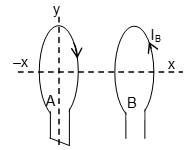(i) Move A to the right along x axis closer to B

(ii) Move A to the left along x axis away from B

(iii) As viewed from above, rotate A clockwise about y axis

(iv) As viewed from above, rotate A anticlockwise about y axis

Which of these actions will induce a current in A only in the direction shown.

1. a. Only (i)
2. b. Only (ii)
3. c. Only (i) and (iv)
4. d. Only (ii) and (iii)

Solution:

The direction of current IB is anticlockwise. The direction of the field due to loop B is towards EMF. It means that magnetic field due to loop A is increased towards right then only this is possible.

Hence, according to lenz law, loop A moves right closer to B.

Question 7: A rigid ball rolls without slipping on a surface shown below.

Which one of the following is the most likely representation of the distance travelled by the ball vs time graph?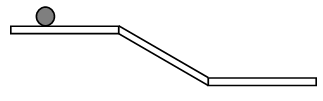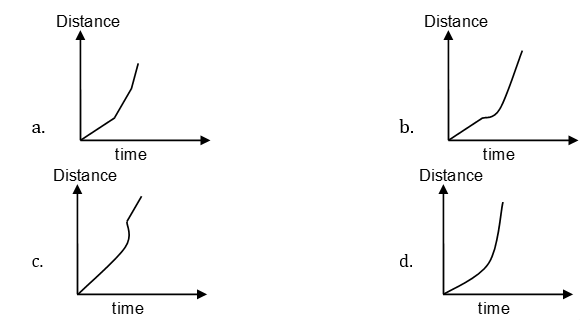Solution:

Since, we know that the slope of distance - time graph gives the velocity of the body.

According to the problem, this is the case of pure rolling.

Now for A to B, pure rolling will have the velocity.

V = r ω = constant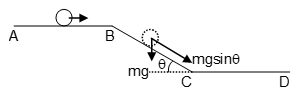So, this uniform pure rolling and in this case no friction will act on the body.

While going B to C, we see that plane is inclined and mg will work downward and mg sin θ will also work down the inclined plane (θ is the angle with horizontal). So, in this case, velocity v will increase therefore r ω will also increase, it means fstatic(static frictional force) ≠ 0

i.e it is the case of accelerated pure rolling.

While going C to D:- v = r ω = constant ⇒ uniform pure rolling

Now, we can say

For A → B slope = constant (as velocity is constant)

B → C slope = increasing (as velocity is increasing)

C → D slope = constant

But slope C → D > slope of A → B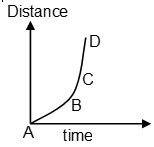Question 8: In an experiment, setup A consists of two parallel wires which carry currents in opposite directions as shown in the figure. A second setup B is identical to setup A, except that there is a metal plate between the wires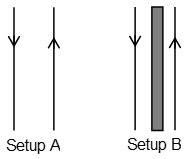Let, FA and FB be the magnitude of the force between the two wires in setup A and setup B, respectively.

1. a. FA > FB ≠ 0
2. b. FA < FB
3. c. FA = FB ≠ 0
4. d. FA > FB = 0

Solution:

Since, force per unit length is given by (when current flows through the two parallel wires)

$\frac{dF}{dl} = \frac{\mu_o}{4 \pi}\frac{2I_1I_2}{r}$

μ0 → permeability of vacuum

In setup B, a metal plate is placed due to which metal may get magnetized and it may also exert force on current carrying wire but force between two wires remain same however, net force on wire may get changed due to the magnetic field produced by magnetized metal.

Question 9: In the circuit, wire 1 is of negligible resistance, then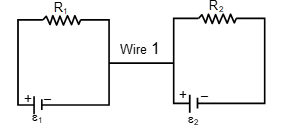1. a. Current will flow through wire 1 if ε1 ≠ ε2
2. b. Current will flow through wire 1 if ε1 /R1 ≠ ε2/R2
3. c. Current will flow through wire 1 if (ε1 + ε2)/(R1+ R2) ≠ (ε1 - ε2)/ (R1–R2)
4. d. No current will flow through wire 1.

Solution: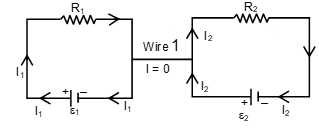Let, the current in first loop through EMF ε1 is I1 and in second loop through EMF ε2 is I2. Whatever the charge leaves this battery of EMF ε1 in unit time, the same amount of charge enters this battery in unit time and same thing is for battery of EMF ε2

Current through the wire 1 is zero.

[It follows the Kirchoff’s loop rule junction rule.]

Question 10: The radius of a nucleus is given by r0 A1/3 where r0 = 1.3 × 10–15 m and A is the mass number of the nucleus, the Lead nucleus has A = 206 the electrostatic force between two protons in this nucleus is approximately

1. a. 102N
2. b. 107 N
3. c. 1012N
4. d. 1017N

Solution:

Given:- radius of a nucleus: r = r0 A1/3

r0 = 1.3 × 10-15 m.

A = mass number of nucleus which is given 206

Let us assume that the two protons are situated at the periphery of the nucleus of radius r.

So, the electrostatic force between them is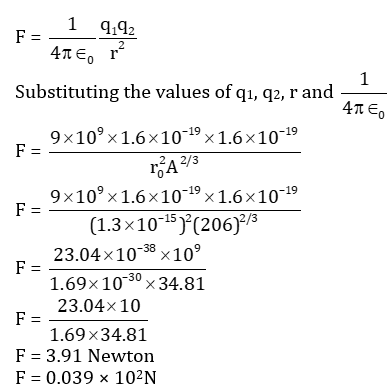Question 11: A hollow lens is made of thin glass and in the shape of a double concave lens. It can be filled with air, water of refractive index 1.33 or CS2 of refractive index 1.6. It will act as a diverging lens if it is

1. a. filled with air and immersed in water.
2. b. filled with water and immersed in CS2.
3. c. filled with air and immersed in CS2.
4. d. filled with CS2 and immersed in water.

Solution:

Given:- A hollow lens made of thin glass in shape of double concave lens.

It is filled with air and for air, refractive index is

μair = 1

μwater = 1.33

μcs2 = 1.6(A) n2 → air = 1

n1 → water = 1.33

Clearly, n2 < n1 ⇒ It will not act as a diverging lens.

(B) n2 = 1.33

n1 = 1.6

Clearly, n2 < n1 ⇒ It will not act as a diverging lens.

(C) n2 = 1

n1 = 1.6

Clearly, n2 < n1 ⇒ it will not act as a diverging lens.

(D) n2 = 1.6

n1 = 1.33

Clearly, n2 > n1

So, it will act as a diverging lens.

Question 12: A stone thrown down with a speed u takes a time t1 to reach the ground, while another stone, thrown upwards from the same point with the same speed, takes time t2. The maximum height the second stone reaches from the ground is

1. a. (1/2) gt1 t2
2. b. g/8 (t1 + t2)2
3. c. g/8 (t1 – t2)2
4. d. 1/2 gt22

Solution:

Here, we are considering the two cases for stone 1 and for stone 2. In both the cases, we consider all vector quantities positive in upward direction and negative in downward direction.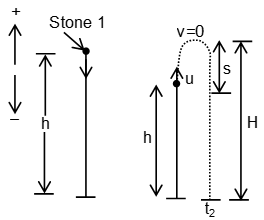For stone 1, let the thrown speed be u and time taken to reach the ground be t1 and height taken be h.

For stone 2, from same point, it is thrown in upward direction with same speed and let the taken to reach the ground be t2 then we have to find maximum height of stone 2 i.e. H = h + s.

For stone 2: - At the maximum height velocity (v) will be zero.

From 1st equation of motion v = u + at

0 = u + at

t = u/g (a = -g downwards) …..(1)

And from 3rd equation of motion,

v2 – u2 = 2as

0 – u2 = 2(–g)s

s = u2/2g ……(2)

For stone 1: Applying 2nd equation of motion,

s = ut + (1/2)at2

Substituting the values,

–h = – ut1 + 1/2(–g)t12

h = ut1 + ½ gt12 …….(3)

For stone 2:- Applying 2nd equation of motion

s = ut + at2

Substituting the values,

–h = ut2 + (–g) t22 ………(4)

Adding equation (1) and equation (2),

0 = u (t1 + t2) + (1/2)g (t12 – t22)

u (t1 + t2) = (1/2)g (t22 – t12)

u (t1 + t2) =(1/2) g(t2 – t1) (t2 + t1)

u = (g/2) (t2 – t1) ………(5)

Multiplying equation (1) by t2 and equation (2) by t1 and solving,

h = (1/2) gt1t2 ….(6)

Since, maximum height H = h + s = H

Substituting the value of u and h from equation (5) and (6), we get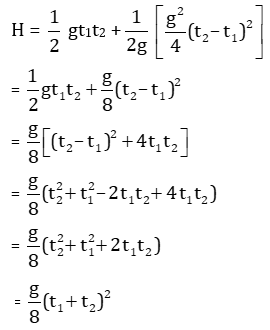Question 13: An electric field due to a positively charged long straight wire at a distance r from it is proportional to r–1 in magnitude. Two electrons are orbiting such a long straight wire in circular orbits of radii 1 Å and 2 Å. The ratio of their respective time periods is.

1. a. 1 : 1
2. b. 1: 2
3. c. 2 : 1
4. d. 4 : 1

Solution:

Let us consider, a long straight wire having some positive charge; electrons at distance r from it and are revolving around it.

So, electric field at distance r (for long straight wire) is given by

E = K/r, K =constant

The force experienced by the electron acts as centripetal force and its direction is towards the centre.

Fe = Fc ; Fe = force experienced by the electron and Fc = centripetal force

eE = mr ω2 ;m = mass of the electron and ω = angular velocity

Since ω = 2π/T,

e(K/r) = mr (4π2/T2)

or T = 2π r √(m/eK)

Because √ (m/eK) is constant therefore T ∝ r

T1/T2 = r1/r2 = 1/2

T1 : T2 = 1:2

Question 14: Two particles of identical mass are moving in circular orbits under a potential given by V(r) = Kr-n, where K is a constant. If the radii of their orbits are r1, r2 and their speeds are v1, v2, respectively, then

1. a. v12r1n = v22r2n
2. b. v12r1-n = v22r2-n
3. c. v12r1 = v22r2
4. d. v12r12-n = v22r22-n

Solution:

Given:- Potential of circular orbit v(r) = Kr–n; K = constant

Radii of orbits = r1 and r2

Speed of particles = v1 and v2

We know that gravitational potential in this case is potential energy (U) per unit mass (m) i.e.

V = U/m ⇒ U = mV = mKr–n

For a conservative force, F = – dU/dr = – d/dr (mKr–n)

= mK d/dr (r –n)

= mn Kr –n–1

F = mnK/rn+1

Now this force is going to act as centripetal force.

F = Fcentripetal

mnk/rn+1 = mv2/r

nK = v2 (rn+1 /rn) ⇒ v2rn =nK = constant

Therefore, v12 r1n = v22 r2n

Question 15: Mercury is often used in clinical thermometers. Which one of the following properties of mercury is not a reason for this?

1. a. The coefficient of the thermal expansion is large.
2. b. It is shiny
3. c. It is a liquid at room temperature.
4. d. It has high density.

Solution:

Most metals are good conductors of heat and they are solids at room temperature. Mercury is the only one in liquid state at room temperature. It’s used in thermometers because it has high coefficient of expansion.

Hence, the slightest change in temperature is notable when it’s used in a thermometer. It also has a high boiling point which makes it very suitable to measure higher temperature. Also, it has shiny appearance and doesn’t stick to the glass surface of glass.

So, high density is not the reason for its uses in clinical thermometers

Question 16: Which one of the following four graphs best depict the variation with x of the moment of inertia I of a uniform triangular lamina about an axis parallel to its base at a distance x from it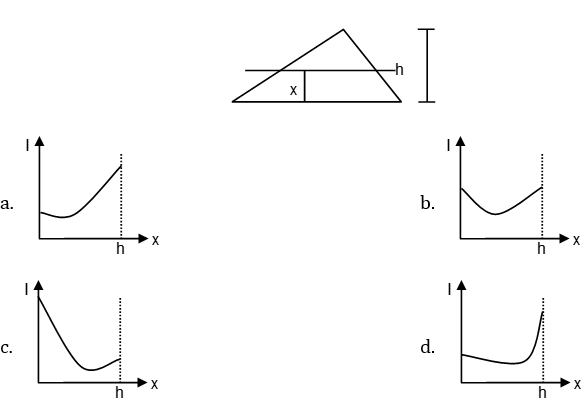Solution: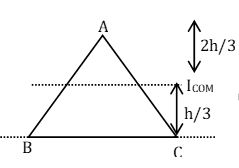(Moment of inertia passing through the centre of mass)

Following the parallel axis theorem,

I = ICOM + Mx2

Moment of inertia is minimum at the centre of mass axis.

So, the conclusion is as we go close to the centre of mass, moment of inertia decreases and further after reaching the centre of mass, moment of inertia continuously goes on increasing.

Question 17: A rectangular block is composed of three different glass prisms (with refractive indices μ1, μ2 and μ3) as shown in the figure below. A ray of light incident normal to the left face emerges normal to the right face. Then the refractive indices are related by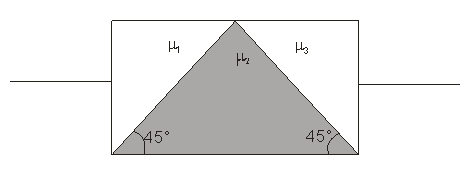1. a. μ12 + μ22 = 2μ32
2. b. μ12 + μ22 = μ32
3. c. μ12 + μ32 = 2μ22
4. d. μ22 + μ32 = 2μ12

Solution: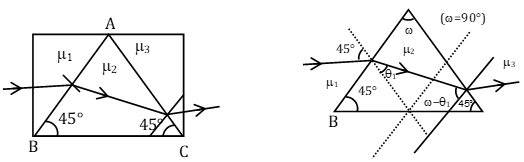Applying Snell’s law:-

i.e. μ = sin i/sin r = μ21

where i = angle of incidence, r = angle of refraction

For surface AB:-

μ1 sin45° = μ2 sin θ1 ; { i = 45°, sin 45° = 1/√2 , r = θ1}

μ1 (1/√2) = μ2 sin θ1 ……..(1)

For surface AC:-

μ2 sin (ω - θ1) = μ3 sin45°

As, ω = 90 {from triangle law 45° + 45° + ω = 180° so, ω = 90°}

Substituting the value of ω,

μ2 sin (90 – θ1 ) = μ3 sin45°

μ2 cosθ1 = μ3(1/√2) …….(2)

Squaring and adding equation (1) and (2), we get

μ12 + μ32 = 2μ22

Question 18: A uniform metal plate shaped like a triangle ABC has a mass of 540 gm. the length of the sides AB, BC and CA are 3 cm, 5 cm and 4 cm, respectively. The plate is pivoted freely about the point A. What mass must be added to a vertex, so that the plate can hang with the long edge horizontal?

1. a. 140 gm at C
2. b. 540 gm at C
3. c. 140 gm at B
4. d. 540 gm at B

Solution: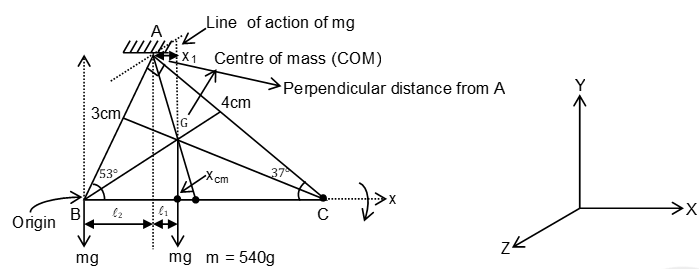We know that for a triangle, the centre of mass is located at centroid. At the centroid G,.force mg will act downward and due to it, a torque is produced about an axis passing through A. Clearly, we can see that mg x x1 will produce a torque that will try to make point C in clockwise direction.

To keep it in equilibrium a mass M is hung at point B.

Now for the rotational equilibrium,

Torque produced by M should be equal to torque produced by m.

τM = τm ….(1)

Now coordinates of centroid (COM) of triangle,

xcm = (x1+x2+x3)/3

Let Point B be the origin.

For B:

x1 = 0

For A:

x2 = 3 cos (53o) = 3 x 0.6

For C:

x2 = 5 cm

Therefore, xcm = (0+3x0.6 + 5)/3 = 6.8/3

From figure,

l1 = xcm – l2

Here l2 = 3 cos(530) = 1.8

So, l1 = 1.4/3 cm

Now from eq (1),

Mg l2 = mg l1

M x 1.8 = 540 x 1.4/3

M=140 g

Question 19: A 20 gm bullet whose specific heat is 5000 J / (kg–°C) and moving at 2000 m/s plunges into a 1.0 kg block of wax whose specific heat is 3000 J /(kg –°C). Both bullet and wax are at 25°C and assume that (i) the bullet comes to rest in the wax and (ii) all its kinetic energy goes into heating the wax. Thermal temperature of the wax in °C is close to

1. a. 28.1
2. b. 31.5
3. c. 37.9
4. d. 42.1

Solution:

Given:-

Mass of the bullet m1 = 20g

Velocity of the bullet v = 2000 m/s

Mass of the wax m2 = 1kg

Specific heat of wax = 3000 j/kgoC

Specific heat of bullet = 5000 J/kgoC

Both bullet and wax are at 25oC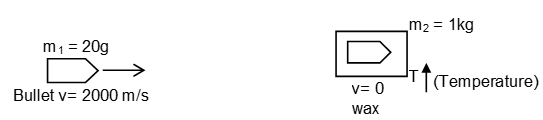When bullet plunged into wax, it comes into rest and temperature of system will increase.

By principle of conservation of energy, we can say that

Loss in kinetic energy of bullet = Heat gained by bullet wax system.

(1/2) mv2 = m1 s1 ΔT + m2 s2 Δ T

Substituting the values, we get

(1/2) x 20/103 x (2000)2 = (20/103 x 5000 + 1 x 3000)(T-25)

On solving above equation, we have

T = 37.9oC

Question 20: A"V" shaped rigid body has two identical uniform arms. What must be the angle between the two arms so that when the body is hung from one end, the other arm is horizontal?

1. a. cos–1 (1/3)
2. b. cos–1 (1/2)
3. c. cos–1 (1/4)
4. d. cos–1 (1/6)

Solution: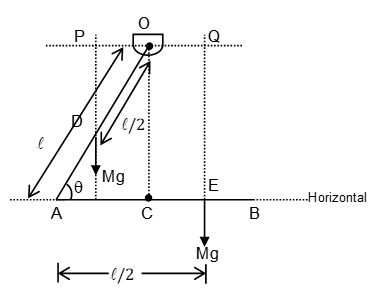Since body is identical, so mass of both the arms will be same i.e. M.

If we consider rotational equilibrium of V–shaped body, the net torque about O (τnet) must be zero.

For this OP = OQ …….(1)

From triangle OPD,

PQ/(l/2) = cos θ ⇒ PQ = (l/2) cos θ

From eq(1)

⇒ OQ = (l/2) cosθ

From figure, AE = AC + CE

⇒ AE = l cos θ + OQ

⇒ (l/2) = l cosθ + (l/2) cos θ

⇒ cos θ = 1/3

Or θ = cos-1(1/3)

### KVPY-SA 2016 Physics Paper with Solutions